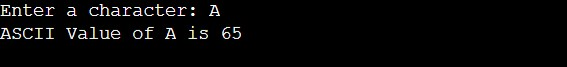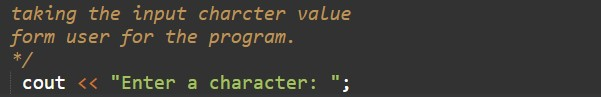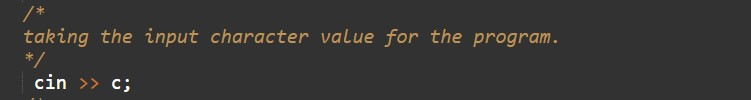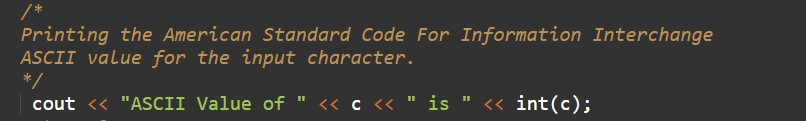# C++ Program to Find ASCII Value of a Character

In this tutorial you will learn about the C++ Program to Find ASCII Value of a Character and its application with practical example.

## C++ Program to Find ASCII Value of a Character

In this tutorial, we will learn to create a C++ program that will find the ASCII Value of a Character in C++ programming.

## Prerequisites

Before starting with this tutorial we assume that you are best aware of the following C++ programming topics:

• Operators in C++ Programming.
• Basic Input and Output function in C++ Programming.
• Basic C++ Programming.

## Program to Find ASCII Value of a Character:-

American Standard Code For Information Interchange stands for ASCII. The ASCII is an encoded format. In c++ programming, we can take the input from the user in character format. In today’s program, we will take input in character format. Secondly, we will find the ASCII Value of a Character the input with the help of a small program. The ASCII values are the most useful code in any programing language to know the characters.

## Output:-In the above program, we have first initialized the required variable.• c = it will hold the integer value of the Remainder.

Taking Input divisor and dividend from the user.Calculating the quotient and the remainder.Printing the output.In this tutorial we have learn about the C++ Program to Find ASCII Value of a Character and its application with practical example. I hope you will like this tutorial.